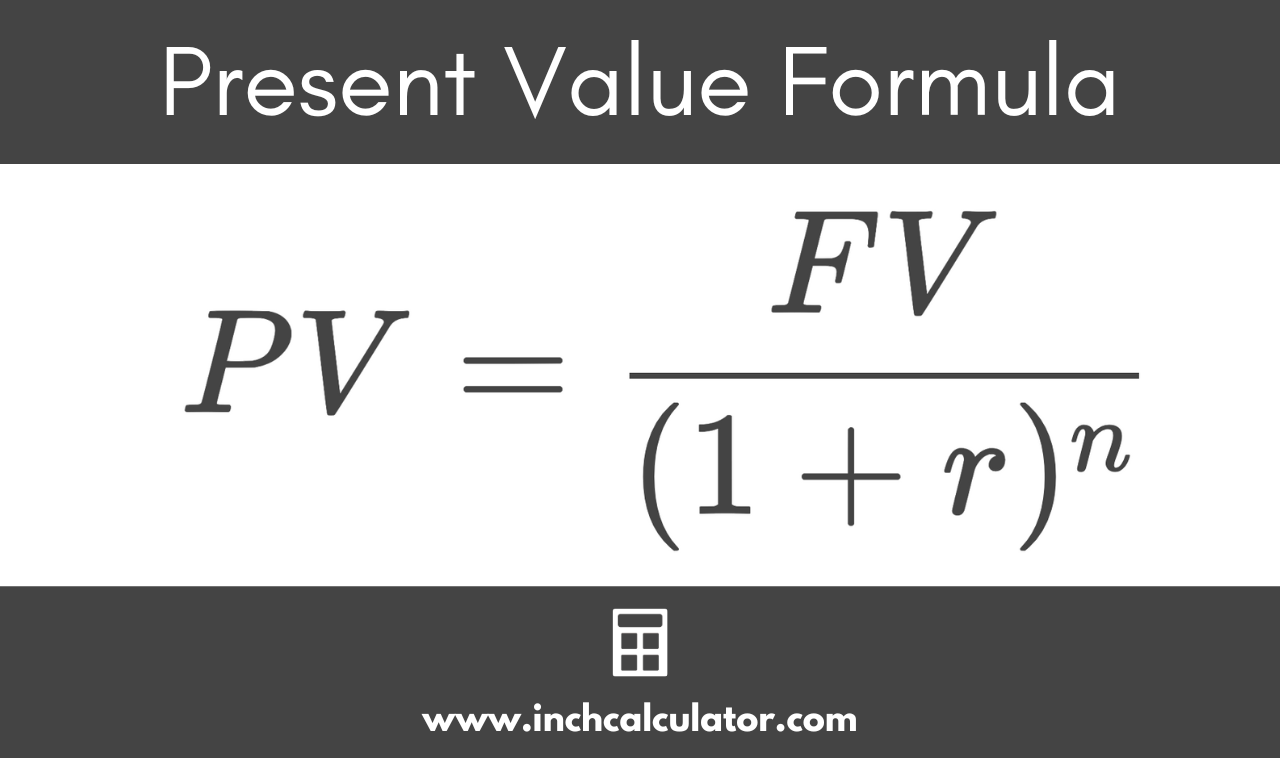# Present Value Calculator

Calculate the present value of an investment given its future value, the rate, and time using the PV calculator below.

$% years ## Results:  Present Value:$729.88 Total Interest: $270.12 This calculation is based on widely-accepted formulas for educational purposes only - this is not a recommendation for how to handle your finances, and it is not an offer to lend or invest. Consult with a financial professional before making financial decisions. Learn how we calculated this below ## On this page: ## How to Calculate Present Value If you receive payments over time, the present value is what those payments are worth today. You can think of present value as the current value of those payments without compound interest accrued. You can also calculate the present value of an investment. If you are investing for a future goal, the present value tells you what you need to invest today to achieve that goal. To calculate present value, enter the future value, interest rate, and time period into the present value calculator above. You can also do this without a calculator using a simple formula. ### Present Value FormulaThe present value formula is: PV = \frac{FV}{(1 + r)^{n}} Where: PV = present value FV = future value r = interest rate n = number of periods The present value PV of an asset or investment is equal to the future value FV divided by 1 plus the interest rate r to the n power, where n is the number of compounding periods. For example, let’s assume you want to retire in 40 years with$2 million. You believe that you can earn an average rate of return of 10% over this timeframe. How much will you need to invest today to meet your retirement goals?

PV = \frac{\$2,000,000}{(1 + 0.1)^{40}} PV = \frac{\$2,000,000}{1.1^{40}}
PV = \frac{\$2,000,000}{45.25926} PV = \$44,189.86

You would need to invest a little more than $44,000 to end up with$2 million at the end of 40 years, assuming an average rate of return of 10%. As the present value calculator shows, almost all the growth is due to interest received.

## How to Find the Present Value of an Annuity

An annuity is a financial contract that promises to make future payments to the annuity holder. The present value of an annuity can be calculated using our annuity calculator (under the present value tab).

You can also apply the present value of an annuity formula to calculate it yourself.

### Present Value of an Annuity Formula

The formula to calculate the present value of an annuity is:

PV = PMT \times \left [ \frac{1 − (1 + i)^{−n}}{i} \right ]

Where:
PV = present value of an ordinary annuity
PMT = payment amount
i = interest rate
n = number of payments

For example, let’s assume you will receive an annuity payment of $2,000 each year for 5 years at an annual interest rate of 6.5%. PV = \$2,000 \times \left [ \frac{1 − (1 + 0.065)^{−5}}{0.065} \right ]
PV = \$2,000 \times \left [ \frac{1 − 1.065^{−5}}{0.065} \right ] PV = \$2,000 \times \left [ \frac{1 − 0.7299}{0.065} \right ]
PV = \$2,000 \times \left [ \frac{0.2701}{0.065} \right ] PV = \$2,000 \times 4.156
PV = \$8,310.77 So, the present value of this annuity is equal to$8,310.77.

You can also use our present value of an annuity calculator, which accounts for annual payments.

### Why is present value important?

Present value (and the time value of money as a whole) plays an important role in many financial decisions since it is a method of determining the value of an investment and deciding what a fair price is for it.

Individuals use present value to forecast how much they will need to invest today to meet retirement goals, businesses use it when deciding to invest in capital, and investors use it to value stocks or bonds.

### How is present value different from future value?

The present value is what a future stream of payments is worth today, whereas the future value is what those streams of payments are worth at some point in the future.

Assuming there is a positive interest rate, the future value will be higher than the present value since dollars today are worth more than dollars in the future due to the time value of money. Thus, a higher dollar amount is needed in the future to equal a specific dollar amount today.

### Is present value the same as net present value?

Net present value (NPV) is a tool used in corporate finance and capital budgeting to value a potential investment opportunity.

NPV is similar to the present value formula shown above in that they both calculate the value of discounted cash flows, but NPV is the difference between the cash flows of the investment and the costs of the investment.

So, when calculating NPV you would subtract the initial investment amount, while this is not part of the PV calculation.